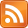# Reanimator Ltd

## High-performance coding by Eddie Edwards

13 Feb 2019, 15:55 UTC

A normal map contains RGB values representing normals (Nx,Ny,Nz).

Tangent space is defined as T = dP/du, B = dP/dv, N = dP/dd where u,v are the texture coordinates, P is position in world space, and d is distance from the surface. By definition, N.T = 0 and N.B = 0, but T.B may not be zero.

This definition of tangent space gives:

``````dP = du.T + dv.B + dd.N
``````

Where du,dv are changes in U,V coordinates, dd is change in depth, and dP is change in worldspace position.

So one might think we should transform a normal from a normal map as follows:

``````N' = Nx.T + Ny.B + Nz.N
``````

However, this does not necessarily have the desired effect. If T and B are skewed, normals are not skewed in the expected way, *if* by expected we mean corresponding to a warping of an underlying heightmap which the normals merely represent.

By that definition, the normal is actually the cross product of two heightfield vectors:

``````N = (c,0,-a) ^ (0,d,-b) = (ad,bc,cd)
``````

Here, -a and -b represent the change in height across a texel, and c and d are normalizing factors.

These two vectors can be transformed by the TBN matrix as follows.

``````N' = (c.T - a.N)^(d.B - b.N)
= cd.T^B + ad.B^N + bc.N^T + ab.N^N
= cd.T^B + ad.B^N + bc.N^T
``````

In terms of the original normal this gives us:

``````N' = Nx.B^N + Ny.N^T + Nz.T^B
``````

Note that N and T^B can be recovered from B^N and N^T, so we only have to store these two vectors. Then we can use some vector identities to solve for N and T^B as follows:

``````A^(B^C) = (A.C)B - (A.B)C
(A^B).(C^D) = (A.C)(B.D) - (B.C)(A.D)
(A^B).C = (B^C).A = (C^A).B
``````

Using these we can show some results:

``````N^(T^B) = (N.B)T - (N.T)B = 0
``````

Since the tangent plane is perpendicular to the normal, the normal is parallel to T^B. In particular:

``````T^B = sN
``````

Now we can show that the cross product of the two vectors we will store is exactly T^B:

``````(B^N)^(N^T) = ((B^N).T)N - ((B^N).N)T
= ((B^N).T)N
= ((T^B).N)N
= (sN.N)N
= sN
= T^V
``````

Note that we have never used the result that |N| = 1. In fact |N| can represent a bump scale: |N| -> 2|N| makes the bumps twice as high. If |T| and |B| are greater than one, |N| staying at one means the bumps stay the same height as the image stretches - so the embossing is shallower. |N| keeping pace with |T| and |B| means the bumps scale up with the stretching, so the overall normals are unchanged. Thus overall,

• We compute the normal N at each vertex
• We compute the tangents T,B at each vertex, s.t. T.N = B.N = 0
• We compute the vectors B^N and N^T, and store these (including magnitude)
• We reconstruct T^V = (B^N)^(N^T)
• We scale B^N and N^T by BumpScale, to reflect scaling N by BumpScale
• We can reconstruct N = normalize(T^V) for external purposes, if we like
• If BumpScale is not specified for a model, we can use some kind of average of |T| and |B| over the whole mesh; we can't assume |T| or |B| will be anything like 1